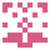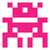# 2067. Building Roads1，用 克鲁斯卡尔 做的同学一定要记得开大数组， 点有1000个，那么你开至少 1000*1000/2 的空间用来存边（因为你是对所有点进行了两次循环加入边），一般是 struct 一个 Edge 这种。

2， 题目里面点的坐标是整数，如果你设置的 struct POINT 里面是用的 int xx,yy; 那么你在计算 两个点之间距离 的时候，如果按照 sqrt((po[i].x-po[j].x)(po[i].x-po[j].x) + (po[i].y-po[j].y)(po[i].y-po[j].y)) 就可能出错。因为题目坐标是最大到(1千万，1千万)。 那么这点到原点的距离，用前面那个式子是没有类型转换的，就不行的，所以设置为 double xx,yy; 是可以的。另外一种就是不要用前面这个式子，而用 sqrt(pow(po[i].x-po[j].x, 2.0) + pow(po[i].y-po[j].y, 2.0))；### define maxn 1000000

using namespace std;

int pre;

void init(int n){
for(int i=1;i<=n;i++)
pre[i]=i;
}

int find(int x){
while(x!=pre[x])
x=pre[x];
return x;
}

void join(int x,int y){
int fx=find(x);
int fy=find(y);
if(fx!=fy)
pre[fx]=fy;
}

struct Node{
double x;
double y;
}node;

struct Edge{
int u;
int v;
double cost;
}edge[maxn];

double count_dis(Node a,Node b){
return sqrt((a.x-b.x)(a.x-b.x)+(a.y-b.y)(a.y-b.y));
}

bool cmp(Edge a,Edge b){
return a.cost<b.cost;
}

void solve(int m){
sort(edge,edge+m, cmp);
double re=0;
for(int i=0;i<m;i++){
Edge t=edge[i];
if(find(t.u)!=find(t.v)){
re+=t.cost;
join(t.u, t.v);
}
}
printf(“%.2lf\n”,re);
}

int main(int argc, const char * argv[]){
int n,m;
scanf(“%d%d”,&n,&m);
for(int i=0;i<n;i++){
scanf(“%lf%lf”,&node[i].x,&node[i].y);
}
init(n);
int t=0;
for(int i=0;i<n-1;i++){
for(int j=i+1;j<n;j++){
edge[t].u=i+1;
edge[t].v=j+1;
edge[t++].cost=count_dis(node[i], node[j]);
}
}
while(m–){
int a,b;
scanf(“%d%d”,&a,&b);
join(a, b);
}
solve(t);
return 0;
}